Next: THE FAST FOURIER TRANSFORM Up: Transforms Previous: SAMPLED DATA AND Z

# Z-TRANSFORM TO FOURIER TRANSFORM

We have defined the Z transform as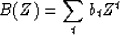(11)
If we make the substitution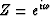we have a Fourier sum''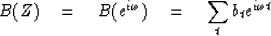(12)
This is like a Fourier integral, and we could do a limiting operation to make it into an integral. Another point of view is that the Fourier integral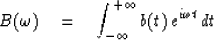(13)
reduces to the sum (12) when b(t) is not a continuous function of time but is defined as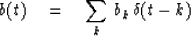(14)
whereis an impulse function.

In the last section we saw that to multiply two polynomials the coefficients must be convolved. The same process in Fourier transform language is that a product in the frequency domain corresponds to a convolution in the time domain.

Although one thinks of a Fourier transform as an integral which may be difficult or impossible to do, the Z transform is always easy, in fact trivial. To do a Z transform one merely attaches powers of Z to successive data points. When one has B(Z) one can refer to it either as a time function or a frequency function, depending on whether one graphs the polynomial coefficients or if one evaluates and graphs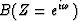for various frequencies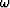.Notice that asgoes from zero to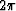,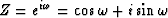migrates once around the unit circle in the counterclockwise direction.

If taking a Z transform amounts to attaching powers of Z to successive points of a time function, then the inverse Z transform must be merely identifying coefficients of various powers of Z with different points in time. How can this simple identification of coefficients'' be the same as the apparently more complicated operation of inverse Fourier integrals? The inverse Fourier integral is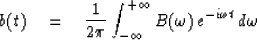(15)

First notice that the integration of Zn about the unit circle or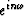over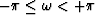gives zero unless n = 0 because cosine and sine are oscillatory; that is,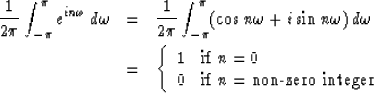(16)
In terms of our discretized time functions, the inverse Fourier integral (15) is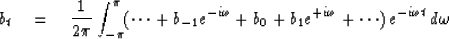(17)
Of all the terms in the integrand (17) we see by (16) that only the term with bt will contribute to the integral; all the rest oscillate and cancel. In other words, it is only the coefficient of Z to the zero power which contributes to the integral, reducing (17) to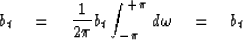(18)
This shows how inverse Fourier transformation is just like identifying coefficients of powers of Z.

In this book and many others, it is common to assume that the time span between data samples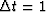is unity. To adapt given equations to other values of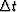,one only need replaceby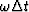;that is,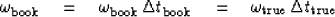(19)

With Z transforms we have the spectrum given on a range offor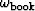.In the limit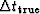goes to zero,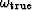has the same infinite limits as the Fourier integral.

When a continuous function is approximated by a sampled function, it is necessary to take the sample spacingsmall enough. The basic result of elementary texts is that, if there is no appreciable energy in a Fourier transform for frequencies higher than some frequency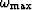,then there is no appreciable loss of information if the sampled at least two points per wavelength. Figure 6 shows how insufficient sampling of a sine wave often causes it to appear as a sine wave of lower frequency.

 1-7a Figure 6 Subsampled sinusoid.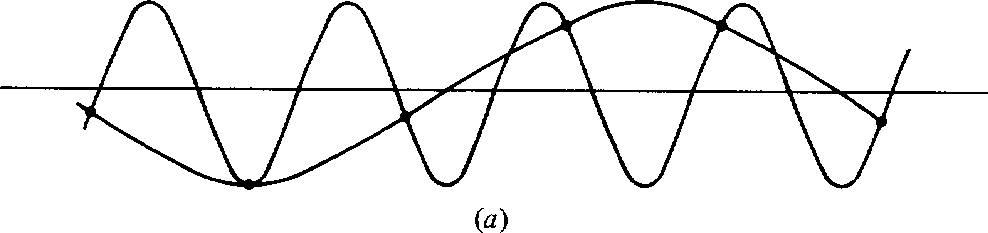If a high-frequency sinusoid is sampled insufficiently often, it becomes indistinguishable from a lower-frequency sinusoid. For this reason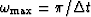is said to be the folding frequency, as higher frequencies are folded down to look like lower frequencies.

In practice, quasi-sinusoidal waves are always sampled more frequently than twice per wavelength.

Next we wish to examine odd/even symmetries to see how they are affected in Fourier transformation. The even part et of a time function bt is defined as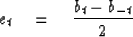(20)

The odd part is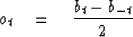(21)
A function is the sum of its even and odd parts. By adding (1-2-10) and (1-2-11), we get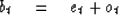(22)

Consider a simple, real, even time function such as (b-1, b0, b1) = (1, 0, 1). Its transform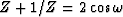is an even function ofsince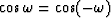.Consider the real, odd time function (b-1, b0, b1) = (-1, 0, 1). Its transform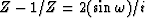is imaginary and odd, since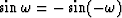.Likewise, the transform of the imaginary even function (i, 0, i) is the imaginary even function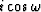and the transform of the imaginary odd function (-i, 0, i) is real and odd. Let r and i refer to real and imaginary, e and o refer to even and odd, and lower-case and upper-case refer to time and frequency functions. A summary of the symmetries of Fourier transformation is shown in Figure 7.

 1-7b Figure 7 Mnemonic table illustrating how even/odd and real/imaginary properties are affected by Fourier transformation.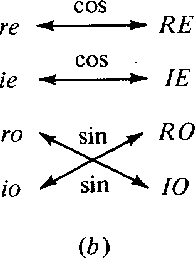More elaborate time functions can be made up by adding together the two point functions we have considered. Since sums of even functions are even, and so on, the table of Figure 7 applies to all time functions. Note that an arbitrary time function takes the form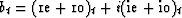.On transformation of bt, each of the four individual parts transforms according to the table

## EXERCISES:

1. Normally a function is specified entirely in the time domain or entirely in the frequency domain. When one is known, the other is determined by transformation. Now let us give half the information in the time domain by specifying that bt = 0 for t < 0, and half in the frequency domain by giving the real part RE + RO in the frequency domain. How can you determine the rest of the function?Next: THE FAST FOURIER TRANSFORM Up: Transforms Previous: SAMPLED DATA AND Z
Stanford Exploration Project
10/30/1997